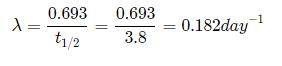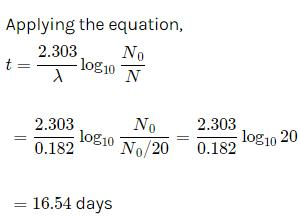Courses

# Test: Nuclei (CBSE Level)

## 30 Questions MCQ Test Physics Class 12 | Test: Nuclei (CBSE Level)

Description
This mock test of Test: Nuclei (CBSE Level) for Class 12 helps you for every Class 12 entrance exam. This contains 30 Multiple Choice Questions for Class 12 Test: Nuclei (CBSE Level) (mcq) to study with solutions a complete question bank. The solved questions answers in this Test: Nuclei (CBSE Level) quiz give you a good mix of easy questions and tough questions. Class 12 students definitely take this Test: Nuclei (CBSE Level) exercise for a better result in the exam. You can find other Test: Nuclei (CBSE Level) extra questions, long questions & short questions for Class 12 on EduRev as well by searching above.
QUESTION: 1

Solution:
QUESTION: 2

Solution:
QUESTION: 3

### Plutonium decays with a half-life of 24000 years. If the plutonium is stored for 72000 years, then the fraction of plutonium that remains is

Solution:
QUESTION: 4

In a nuclear reaction which of the following is conserved

Solution:
QUESTION: 5

Cadmium rods are used in a nuclear reactor for

Solution:

In nuclear reactors, fission of uranium is carried out by bombarding  a slow moving neutron on uranium which results in emission of 3 more neutrons along with the byproducts  So, cadmium rods are used to absorb extra neutrons in order to achieve controlled chain reaction.

QUESTION: 6

In what units is mass measured on the atomic scale?

Solution:
QUESTION: 7

Energies associated with nuclear processes are

Solution:
QUESTION: 8

B210has a half life of 5 days. The time taken for seven-eighth of a sample to decay is

Solution:
QUESTION: 9

If 10% of a substance decays in 10 days, then approximate percentage of substance left after 24 days is

Solution:
QUESTION: 10

Choose the WRONG statement. A thermonuclear fusion reactor is better than a fission reactor for the following reason:

Solution:
QUESTION: 11

A free neutron decays into

Solution:
QUESTION: 12

The disintegration energy is

Solution:
QUESTION: 13

A radioactive substance has a half life of four months. Three fourths of the substance will decay in

Solution:
QUESTION: 14

At a given time there are 25% undecayed nuclei in a sample. After 10 seconds number of undecayed nuclei reduces to 12.5%. Then mean life of the nuclei will be about

Solution:

Half-life of radioactive sample, i.e., the time in which the number of undecayed nuclei becomes half (T) is 10 s.
Mean life, τ=T/loge​2=10s/0.693=1.443×10=14.43s ≈ 15s

QUESTION: 15

Which of the following particles can be added to the nucleus of an atom without changing its chemical properties?

Solution:
QUESTION: 16

Relation between atomic number (Z), neutron number (N) and mass number (A) is

Solution:
QUESTION: 17

α-rays are

Solution:
QUESTION: 18

The half life of radon is 3.8 days. After how many days will only one twentieth of a radon sample be left over?

Solution:Let the initial amount of radon be N0​ and the amount left after t days be N which is equal to N0​/2QUESTION: 19

90% of a radioactive sample is left undisintegrated after time τ has elapsed, what percentage of initial sample will decay in a total time 2τ?

Solution:
QUESTION: 20

The average number of neutrons released by the fission of one uranium atom is

Solution:

Fission also results in the production of several neutrons, typically two or three. On the average, about 2.5 neutrons are released per unit and the energy of a neutron released per fission of a uranium atom are 2 MeV.

QUESTION: 21

The nuclei of isotopes of a given element contain the same number of

Solution:
QUESTION: 22

β -rays and γ-rays are respectively

Solution:
QUESTION: 23

Nuclear fusion is possible

Solution:
QUESTION: 24

What is the main source of energy of the sun?

Solution:
QUESTION: 25

All nuclides with same mass number A are called

Solution:
QUESTION: 26

When a hydrogen bomb explodes, which of the following is used?

Solution:
QUESTION: 27

The half-life T1/2of a radionuclide is

Solution:
QUESTION: 28

A radioactive material decays by simultaneous emission of two particles with respective half lives 1620 and 810 years. The time in years, after which one fourth of the material remains is

Solution:

Since, from Rutherford-Soddy law, the number of atoms left after half-lives is given by
N=N0​(1/2​)n
where, N0​ is the original number of atoms.
The number of half-lives, n= time of decay​/effective half−life
Relation between effective disintegration constant (λ) and half-life (T)
λ=ln2/T​
∴λ1​+λ2​= (​ln2/ T1​)+ ​(ln2/ T2​)
Effective half-life,
1/T​=1/T1​​+1/T2​​=(1/1620)​+(1/810)​
1/T​=1+2/1620 ​⇒T=540yr
∴n=T/540
∴N=N0​(1/2​)t/540⇒N/N0​​=(1/2​)2=(1/2​)t/540
⇒t/540​=2⇒t=2×540=1080yr

QUESTION: 29

The chemical behavior of an atom depends upon

Solution:
QUESTION: 30

Nuclear mass M is found to be

Solution: